Home
Research
Teaching
Jobs / Theses
Software
Other

### Preprints & Work in Progress:

P07c
"Blow-up analysis of fast-slow PDEs with loss of hyperbolicity"

M. Engel and C. Kuehn

P07b
"Graphop mean-field limits for Kuramoto-type models"

M.-A. Gkogkas and C. Kuehn

P06b
"Random attractors via pathwise mild solutions for stochastic parabolic evolution equations"

C.Kuehn, A. Neamtu and S. Sonner

P06a
"Metastable speeds in the fractional Allen-Cahn equation"

F. Achleitner, C.Kuehn, M. Melenk and A. Rieder

P04
"Traveling wave dynamics for Allen-Cahn equations with strong irreversibility"

G. Akagi, C.Kuehn and K.-I. Nakamura

P03b
"Homogenization of fully-coupled chaotic fast-slow systems via intermediate stochastic regularization"

M. Engel, M.-A. Gkogkas and C. Kuehn

P03a
"Travelling waves for discrete stochastic bistable equations"

C. Geldhauser and C. Kuehn

P02d "A universal route to explosive phenomena"

C. Kuehn and C. Bick

P02c
"Multiscale analysis for traveling-pulse solutions to the stochastic FitzHugh-Nagumo equations"

K. Eichinger, M. Gnann and C. Kuehn

P02b
"Numerical continuation for fractional PDEs: sharp teeth and bloated snakes"

N. Ehstand, C. Kuehn and C. Soresina

P02a
"A geometric analysis of the SIR, SIRS and SIRWS epidemiological models"

H. Jardon Kojakhmetov, C. Kuehn, A. Pugliese and M. Sensi

P11d
"Controlling canard cycles"

H. Jardon Kojakhmetov and C. Kuehn

P11c
"A random dynamical systems perspective on isochronicity for stochastic oscillations"

M. Engel and C. Kuehn

P11b
"Predicting sparse circle maps from their dynamics"

F. Krahmer, C. Kuehn and N. Sissouno

P10
"On the influence of cross-diffusion in pattern formation"

M. Breden, C. Kuehn and C. Soresina

P07a
"Discretized fast-slow systems with canard points in two dimensions"

M. Engel, C. Kuehn, M. Petrera and Y. Suris

P11a
"Rough center manifolds"

C. Kuehn and A. Neamtu

 Journal Publications: J76 "Global martingale solutions for quasilinear SPDEs via the boundedness-by-entropy method" G. Dhariwal, F. Huber, A. Jüngel, C. Kuehn and A. Neamtu Annals de l'Institut Henri Poincaré (B) Probabilités et Statistiques, accepted / to appear, 2020 J75 "Coupled dynamics on hypergraphs: master stability of steady states and synchronization"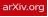R. Mulas, C. Kuehn and J. Jost Physical Review E, Vol. 101, No. 6, 062313, 2020 J74 "Random attractors for stochastic partly dissipative systems"C. Kuehn, A. Neamtu and A. Pein Nonlinear Differential Equations and Applications NoDEA, Vol. 27, No. 35, 2020 J73 "On fast-slow consensus networks with a dynamic weight"H. Jardon Kojakhmetov and C. Kuehn Journal of Nonlinear Science, accepted / to appear, 2020 J72 "Network dynamics on graphops"C. Kuehn New Journal of Physics, Vol. 22, 053030, 2020 J71 "Pathwise mild solutions for quasilinear stochastic partial differential equations"C. Kuehn and A. Neamtu Journal of Differential Equations, Vol. 269, No. 3, pp. 2185-2227, 2020 J70 "Computing invariant sets of random differential equations using polynomial chaos"M. Breden and C. Kuehn SIAM Journal on Applied Dynamical Systems, Vol. 19, No. 1, pp. 577-618, 2020 J69 "Combined error estimates for local fluctuations of SPDEs"C. Kuehn and P. Kürschner Advances in Computational Mathematics, Vol. 46, No. 11, 2020 J68 "Numerical continuation for a fast-reaction system and its cross-diffusion limit"C. Kuehn and C. Soresina SN Partial Differential Equations and Applications, Vol. 1, No. 7, 2020 J67 "Adaptive voter model on simplicial complexes"L. Horstmeyer and C. Kuehn Physical Review E, Vol. 101, No.2, 022305, 2020 J66 "Travelling waves in monostable and bistable stochastic partial differential equations"C. Kuehn Jahresbericht der Deutschen Mathematiker-Vereinigung, Vol. 122, No. 2, pp. 73-107, 2020 J65 "Sample paths estimates for stochastic fast-slow systems driven by fractional Brownian motion"K. Eichinger, C. Kuehn and A. Neamtu Journal of Statistical Physics, Vol. 179, No. 5, pp. 1222-1266, 2020 J64 "Geometry and numerical continuation of multiscale orbits in a nonconvex variational problem"A. Iuorio, C. Kuehn and P. Szmolyan Discrete and Continuous Dynamical Systems S, Vol. 13, No. 4, pp. 1269-1290, 2020 J63 "Random switching near bifurcations"T. Hurth and C. Kuehn Stochastics and Dynamics, Vol. 20, No. 2, 2050008, 2020 J62 "Mathematical analysis of nonlocal  PDEs for network generation"T. Böhle and C. Kuehn Mathematical Modelling of Natural Phenomena, Vol. 14, No. 5, 506, 2019 J61 "Discretized fast-slow systems near pitchfork singularities"L. Arcidiacono, M. Engel and C. Kuehn Journal of Difference Equations and Applications, Vol. 25, No. 7, pp. 1024-1051, 2019 J60 "A survey on the blow-up method for fast-slow systems"H. Jardon Kojakhmetov and C. Kuehn Contemporary Mathematics, AMS, accepted / to appear, 2019 J59 "A gradient flow formulation for the stochastic Amari model"C. Kuehn and J. Tölle Journal of Mathematical Biology, Vol. 79, No. 4, pp. 1227-1252, 2019 J58 "Power network dynamics on graphons"C. Kuehn and S. Throm SIAM Journal on Applied Mathematics, Vol. 79, No. 4, pp. 1271-1292, 2019 J57 "Smoluchowski's coagulation equation with forcing"C. Kuehn and S. Throm Nonlinear Differential Equations and Applications (NoDEA), Vol. 26, No. 3, 17, 2019 J56 "Discretized fast-slow systems near transcritical singularities"M. Engel and C. Kuehn Nonlinearity, Vol. 32, No. 7, pp. 2365-2391, 2019 J55 "Multiscale dynamics of an adaptive catalytic network"C. Kuehn Mathematical Modelling of Natural Phenomena, Vol. 14, No. 4, 402, 2019 J54 "Rigorous validation of stochastic transition paths"M. Breden and C. Kuehn Journal de Mathématiques Pures et Appliquées, Vol. 131, pp. 88-129, 2019 J53 "Duck traps: two-dimensional critical manifolds in planar systems"C. Kuehn and C. Münch Dynamical Systems: An International Journal, Vol. 34, No. 4, pp. 584-612, 2019 J52 "Pattern formation in the doubly-nonlocal Fisher-KPP equation"C. Kuehn and P. Tkachov Discrete and Continuous Dynamical Systems A, Vol. 39, No. 4, pp. 2077-2100, 2019 J51 "Towards sample path estimates for fast-slow stochastic partial differential equations"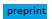M.V. Gnann, C. Kuehn and A. Pein European Journal of Applied Mathematics, Vol. 30, No. 5, pp. 1004-1024, 2019 J50 "Scaling laws and warning signs for bifurcations of SPDEs"C. Kuehn and F. Romano European Journal of Applied Mathematics, Vol. 30, No. 5, pp. 853-868, 2019 J49 "Network topology near criticality in adaptive epidemics"L. Horstmeyer, C. Kuehn and S. Thurner Physical Review E, Vol 98, 042313, 2018 J48 "A note on kernel methods for multiscale systems with critical transitions"B. Hamzi, C. Kuehn and S. Mohamed Mathematical Methods in the Applied Sciences, Vol. 42, No. 3, pp. 907-917, 2018 J47 "Validity of amplitude equations for nonlocal nonlinearities"C. Kuehn and S. Throm Journal of Mathematical Physics, Vol. 59, 071510, 2018 J46 "Analysis and predictability for tipping points with leading-order nonlinear terms"F. Romano and C. Kuehn International Journal of Bifurcation and Chaos, Vol. 28, No. 8, 1850103, 2018 J45 "Early-warning signals for bifurcations in random dynamical systems with bounded noise"C. Kuehn, G. Malavolta and M. Rasmussen Journal of Mathematical Analysis and Applications, Vol. 464, pp. 58–77, 2018 J44 "Tracking particles in flows near invariant manifolds via balance functions"C. Kuehn, F. Romano and H. Kuhlmann Nonlinear Dynamics, Vol. 92, No. 3, pp. 983-1000, 2018 J43 "Stochastic mixed-mode oscillations in a three-species predator-prey model"S. Sadhu and C. Kuehn Chaos: An Interdisciplinary Journal of Nonlinear Science, Vol. 28, No. 3, 033606, 2018 J42 "Quenched noise and nonlinear oscillations in bistable multiscale systems"C. Kuehn EPL (Europhysics Letters), Vol. 120, 10001, 2017 J41 C. Kuehn and C. Münch SIAM Journal on Applied Dynamical Systems, Vol. 16, No. 3, pp. 1650-1685, 2017 J40 "Model spaces of regularity structures for space-fractional SPDEs"N. Berglund and C. Kuehn Journal of Statistical Physics, Vol. 168, No. 2, pp. 331-368, 2017 J39 "Uncertainty transformation via Hopf bifurcation in fast-slow systems"C. Kuehn Proceedings of the Royal Society A, Vol. 473, 20160346, 2017 J38 "Continuation of probability density functions using a generalized Lyapunov approach"S. Baars, J.P. Viebahn, T.E. Mulder, C. Kuehn, F.W. Wubs and H.A. Dijkstra Journal of Computational Physics, Vol. 336, No. 1, pp. 627–643, 2017 J37 "A meeting point of entropy and bifurcations in cross-diffusion herding"A. Jüngel, C. Kuehn and L. Trussardi European Journal of Applied Mathematics, Vol. 28, No. 2, pp. 317-356, 2017 J36 "A dynamical systems approach for the contact-line singularity in thin-film flows"F.B. Belgacem, M.V. Gnann and C. Kuehn Nonlinear Analysis A: Theory, Methods & Applications, Vol. 144, pp. 204-235, 2016 J35 "A numerical framework to understand transitions in high-dimensional stochastic dynamical systems" H.A. Dijkstra, A. Tantet, J. Viebahn, E. Mulder, M. Hebbink, D. Castellane, H. van der Pol, J. Frank, S. Baars, F. Wubs, M. Chekroun and C. Kuehn Dynamics and Statistics of the Climate System, Vol. 1, No. 1, dzw003 (review paper), 2016 J34 "Heterogeneous population dynamics and scaling laws near epidemic outbreaks"A. Widder and C. Kuehn Mathematical Biosciences and Engineering, Vol. 13, No. 5, pp. 1093-1118, 2016 J33 "Regularity structures and renormalisation of FitzHugh-Nagumo SPDEs in three space dimensions"N. Berglund and C. Kuehn Electronic Journal of Probability, Vol. 21, No. 18, pp. 1-48, 2016 [erratum] J32 "A remark on geometric desingularization of a non-hyperbolic point using hyperbolic space"C. Kuehn Journal of Physics: Conference Series, Vol. 727, 012008, 2016 J31 "Numerical continuation and SPDE stability for the 2D cubic-quintic Allen-Cahn equation"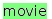C. Kuehn SIAM/ASA Journal on Uncertainty Quantification, Vol. 3, No. 1, pp. 762-789, 2015 J30 "Predictability of critical transitions"X. Zhang, C. Kuehn and S. Hallerberg Physical Review E, Vol. 92, 052905, 2015 J29 "Traveling waves for bistable evolution equations with nonlocal-diffusion"F. Achleitner and C. Kuehn Advances in Differential Equations, Vol. 20, No. 9-10, pp. 887-936, 2015 J28 "Efficient gluing of numerical continuation and a multiple solution method for elliptic PDEs"C. Kuehn Applied Mathematics and Computation, Vol. 266, pp. 656-674, 2015 J27 "Multiscale geometry of the Olsen model and non-classical relaxation oscillations"C. Kuehn and P. Szmolyan Journal of Nonlinear Science, Vol. 25, No. 3, pp. 583-629, 2015 J26 "Early-warning signs for saddle-escape transitions in complex networks"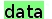C. Kuehn, G. Zschaler and T. Gross Scientific Reports, Vol. 5, 13190, 2015 [download paper + correctly-formatted SI here] J25 "From random Poincaré maps to stochastic mixed-mode-oscillation patterns"N. Berglund, B. Gentz and C. Kuehn Journal of Dynamics and Differential Equations, Vol. 27, No. 1, pp. 83-136, 2015 J24 "Critical slowing down governs the transition to neuron spiking"C. Meisel, A. Klaus, C. Kuehn and D. Plenz PLoS Computational Biology, Vol. 11, No. 2, e1004097, 2015 J23 "Analysis and numerics of travelling waves for asymmetric fractional reaction-diffusion equations"F. Achleitner and C. Kuehn Communications in Applied and Industrial Mathematics, Vol. 6, No. 2, 2015 J22 "On bounded positive stationary solutions for a nonlocal Fisher-KPP equation"F. Achleitner and C. Kuehn Nonlinear Analysis A: Theory, Methods & Applications, Vol. 112, pp. 15-29, 2015 J21 "Early-warning signs for pattern formation in stochastic partial differential equations"K. Gowda and C. Kuehn Communications in Nonlinear Science and Numerical Simulation, Vol. 22, No. 1, pp. 55-69, 2015 J20 "Normal hyperbolicity and unbounded critical manifolds"C. Kuehn Nonlinearity, Vol. 27, No. 6, pp. 1351-1366, 2014 J19 "Critical transitions in social network activity"C. Kuehn, E. Martens and D. Romero Journal of Complex Networks, Vol. 2, No. 2, pp. 141-152, 2014 [see also: OUP Blog] J18 "Large deviations for nonlocal stochastic neural fields"C. Kuehn and M. Riedler Journal of Mathematical Neuroscience, Vol. 4, No. 1, pp. 1-33, 2014 J17 "A mathematical framework for critical transitions: normal forms, variance and applications"C. Kuehn Journal of Nonlinear Science, Vol. 23, No. 3, pp. 457-510, 2013 J16 "Nonlocal generalized models of predator-prey systems"C. Kuehn and T. Gross Discrete and Continuous Dynamical Systems B, Vol. 18, No. 3, pp. 693-720, 2013 J15 "Warning signs for wave speed transitions of noisy Fisher-KPP invasion fronts"C. Kuehn Theoretical Ecology, Vol. 6, No. 3, pp. 295-308, 2013 J14 "Dynamical analysis of evolution equations in generalized models"C. Kuehn, S. Siegmund and T. Gross IMA Journal of Applied Mathematics, Vol. 78, No. 5, pp. 1051-1077, 2013 J13 "Deterministic continuation of stochastic metastable equilibria via Lyapunov equations and ellipsoids"C. Kuehn SIAM Journal on Scientific Computing, Vol. 34, No. 3, pp. A1635-A1658, 2012 J12 "Mixed-mode oscillations with multiple time scales"M. Desroches, J. Guckenheimer, B. Krauskopf, C. Kuehn, H. Osinga and M. Wechselberger SIAM Review, Vol. 54, No. 2, pp. 211-288, 2012 J11 "Time-scale and noise optimality in self-organized critical adaptive networks"C. Kuehn Physical Review E, Vol. 85, No. 2, 026103, 2012 J10 "Hunting French ducks in a noisy environment"N. Berglund, B. Gentz and C. Kuehn Journal of Differential Equations, Vol. 252, No. 9, pp. 4786-4841, 2012 J9 "Scaling effects and spatio-temporal multilevel dynamics in epileptic seizures"C. Meisel and C. Kuehn PLoS ONE, Vol. 7, No. 2, e30371, 2012 J8 "On decomposing mixed-mode oscillations and their return maps"C. Kuehn Chaos: An Interdisciplinary Journal of Nonlinear Science, Vol. 21, No. 3, 033107, 2011 J7 "A mathematical framework for critical transitions: bifurcations, fast-slow systems and stochastic dynamics"C. Kuehn Physica D: Nonlinear Phenomena, Vol. 240, No. 12, pp. 1020-1035, 2011 J6 "Connecting fast-slow systems and Conley index theory via transversality"C. Kuehn Electronic Journal of Differential Equations, Vol. 2010, No. 106, pp. 1-20, 2010 J5 "Homoclinic orbits of the FitzHugh-Nagumo equation: bifurcations in the full system''J. Guckenheimer and C. Kuehn SIAM Journal on Applied Dynamical Systems, Vol. 9, No. 1, pp. 138-153, 2010 J4 "From first Lyapunov coefficients to maximal canards"C. Kuehn International Journal of Bifurcation and Chaos, Vol. 20, No. 5, pp. 1467-1475, 2010 J3 "Computing slow manifolds of saddle-type"J. Guckenheimer and C. Kuehn SIAM Journal on Applied Dynamical Systems, Vol. 4, No. 3, pp. 854-879, 2009 J2 "Homoclinic orbits of the FitzHugh-Nagumo equation: the singular limit''J. Guckenheimer and C. Kuehn Discrete and Continuous Dynamical Systems S, Vol. 2, No. 4, pp. 851-872, 2009 J1 "Scaling of saddle-node bifurcations: degeneracies and rapid quantitative changes"C. Kuehn Journal of Physics A: Mathematical and Theoretical, Vol. 42, No. 4, 045101, 2009

Books:
B2 "PDE Dynamics: An Introduction", C. Kuehn, SIAM, 2019

 PDE Dynamics Christian Kuehn SIAM, 2019

• corrigenda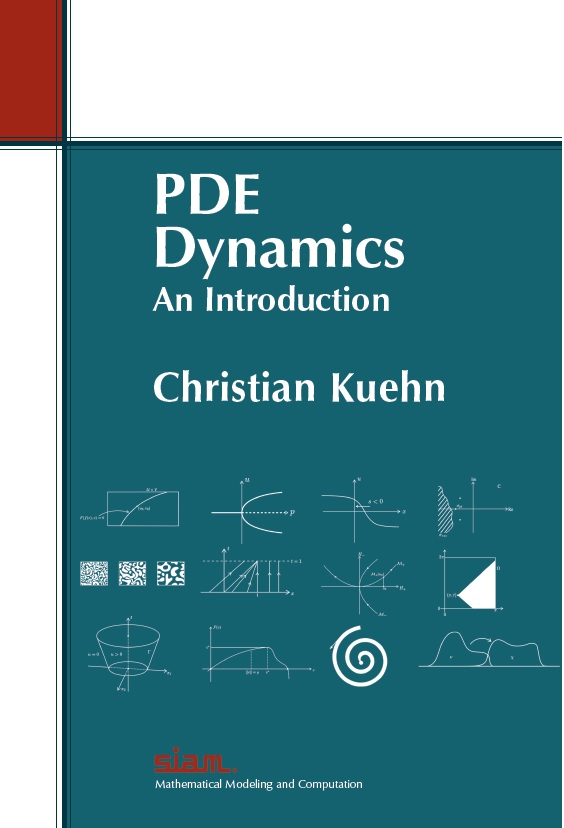B1 "Multiple Time Scale Dynamics", C. Kuehn, Springer, 2015

 Multiple Time Scale Dynamics Christian Kuehn Springer Verlag, 2015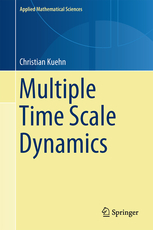Reviews: DSWeb / MathSciNet / SIAM Review / Zentralblatt

 Book Chapters: C2 "Dynamics of stochastic reaction-diffusion equations"C. Kuehn and A. Neamtu in: Finite and Infinite Dimensional Stochastic Equations with Applications to Physics (editors: H. Lisei & W. Grecksch), Wiley, pp. 1-55, 2020 C1 "Moment closure - A brief review"C. Kuehn in: Control of Self-Organizing Complex Systems (editors: E. Schöll, S. Klapp & P. Hövel), Springer, pp. 253-271, 2016

### Further Writings:

L3
"Introduction to Adaptive Network Dynamics" (available upon request)

C. Kuehn

Lecture Notes for: Case Studies in Mathematical Modelling 2018/2019

V2
Book Review of ''Hidden Dynamics'' (by M.R. Jeffrey)C. Kuehn

SIAM Review, Vol. 61, No. 3, within pp. 625–640, 2019.

L2
"Dynamical Systems I: ODEs and Introduction to Nonlinear Dynamics"

C. Kuehn

AMS Open Math Notes, 66pp., 2018

L1
"Dynamical Systems II: A Concise Graduate Course"

C. Kuehn

AMS Open Math Notes, 125pp., 2018

V1
Book Review of "Network Science" (by A.-L. Barabasi)C. Kuehn

SIAM Activity Group, DSWeb Magazine, April 2017

AC
"Addenda and Corrigenda to Journal Publications"

C. Kuehn

T4 "Multiscale dynamical systems: analysis and numerics"
C. Kuehn
Habilitation: Vienna University of Technology, 2016

E1 "The curse of instability"C. Kuehn
Complexity, (section: 'Simply Complex'), Vol. 20, No. 6, pp. 9-14, 2015

T3 "Multiple time scale dynamics with two fast variables and one slow variable"
C. Kuehn
Ph.D. thesis: Cornell University, 2010

T2 "Introduction to potential theory via applications"
C. Kuehn
Part III essay: University of Cambridge, 2006

T1 "Stability analysis of nonlinear subdivision schemes"
C. Kuehn
B.Sc. thesis: Jacobs University, 2005